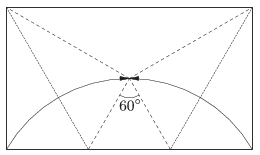Mathematical and Physical Journal
for High Schools
Issued by the MATFUND Foundation
 Already signed up? New to KöMaL?

#Problem C. 1303. (September 2015)

C. 1303. The area of a rectangular origami sheet is $\displaystyle 130~\rm{cm}^2$. The sheet is folded symmetrically by forming a regular triangle with two adjacent vertices turned inwards, as shown in the figure. Find the lengths of the sides of the original sheet.(5 pont)

Deadline expired on October 12, 2015.

### Statistics:

 338 students sent a solution. 5 points: 259 students. 4 points: 53 students. 3 points: 12 students. 2 points: 4 students. 1 point: 2 students. 0 point: 3 students. Unfair, not evaluated: 5 solutionss.

Problems in Mathematics of KöMaL, September 2015